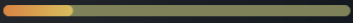# Help with Statistic Bar Scaling

``````local current = 1
local maxVal = 5

button.MouseButton1Click:Connect(function()
Bar.Size = UDim2.new(current / maxVal, 0, 1, 0)
end)
``````

The starting value here is 1, and the scale of the bar goes up like this:But is there a way to retain the same scale with starting values more than 1? (Some of my starting values have a value of 8+.)

I tried putting the current to 8 and the maxVal to 17 but the bar went past instead lol.

1 Like

You need to use the Size of the bar as a point to help get the size it should be

Try this and se if it helps

``````local current = 1
local maxVal = 5

local xSize = Bar.Size.X.Scale --Just so we have the original size

button.MouseButton1Click:Connect(function()
local useSize = (current/maxVal) * xSize --A formula that is useful for this
Bar.Size = UDim2.new(useSize, 0, 1, 0)
end)``````

you can always clamp the current variable

``UDim2.new(math.clamp(current, 0, maxVal) / maxVal, 0, 1, 0)``